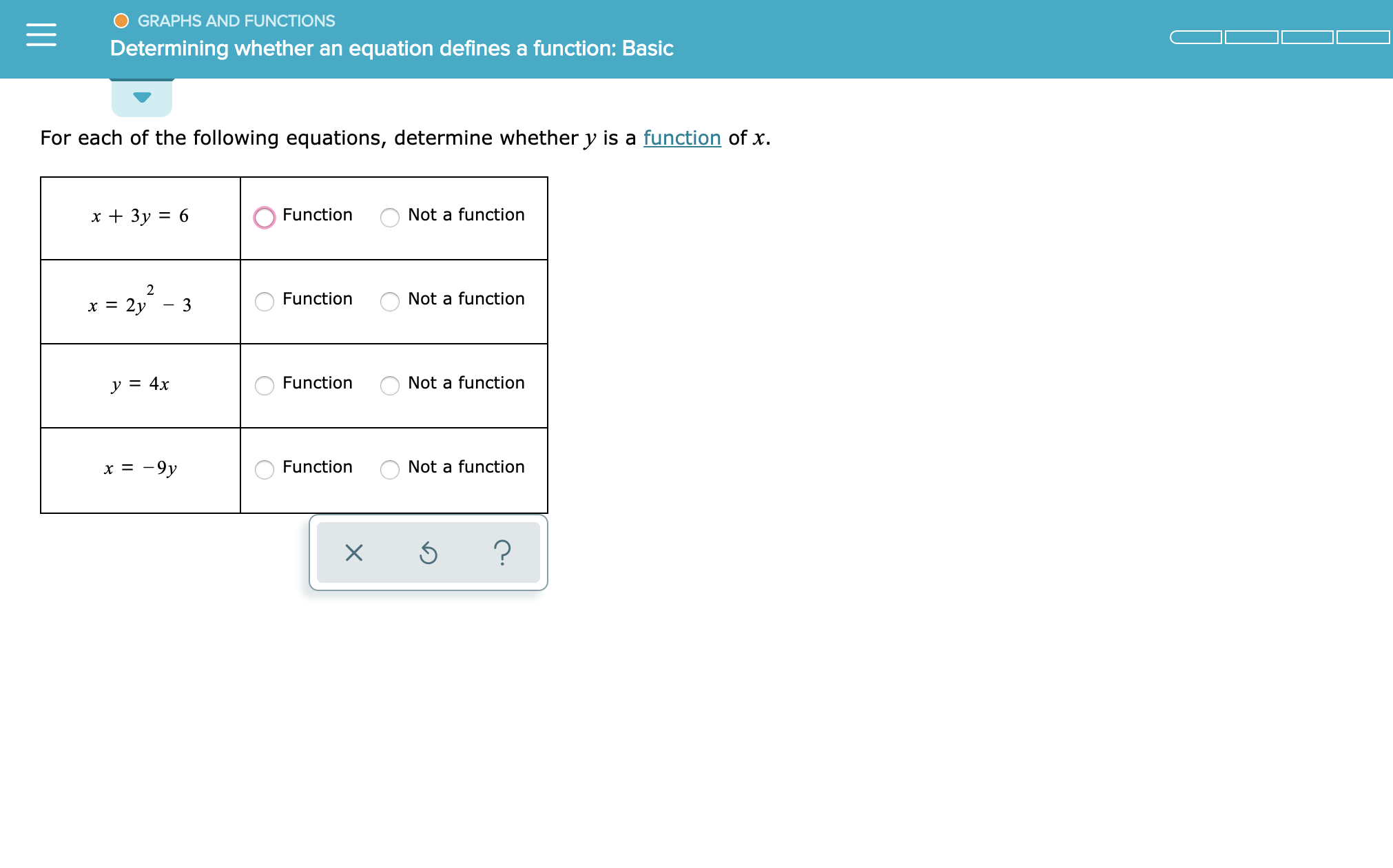# GRAPHS AND FUNCTIONSDetermining whether an equation defines a function: BasicFor each of the following equations, determine whether y is a function of xx3y 6Not a functionFunction2Not a functionFunctionx 2y3=Not a functionFunctiony = 4xx 9yNot a functionFunction?

Question
2 views

See attachedhelp_outlineImage TranscriptioncloseGRAPHS AND FUNCTIONS Determining whether an equation defines a function: Basic For each of the following equations, determine whether y is a function of x x3y 6 Not a function Function 2 Not a function Function x 2y 3 = Not a function Function y = 4x x 9y Not a function Function ? fullscreen
check_circle

Step 1

An equation is a function if there is &nbs...

### Want to see the full answer?

See Solution

#### Want to see this answer and more?

Solutions are written by subject experts who are available 24/7. Questions are typically answered within 1 hour.*

See Solution
*Response times may vary by subject and question.
Tagged in

### Functions